### MNIST digit recognition

Sat, Aug 22, 2020 3-minute read

In this article, I will introduce basic knowledge of Tensorflow to the real example of Tensorflow.

## Maths operations in Tensorflow

### Machine Learning is making a boom in the tech world, specifically for the developers and for the talented data…

#### medium.com

Above article will help you to learn the basic math operations in Tensorflow. It will be good to have work with session and placeholder.

Let’s move to the

We have 28*28 = 784 pixels of the images. In training.csv we have the correct digit of the number. We will create a model based on the training.csv file and will submit the output of the predicted values on testing.csv file.

# seperating labels and images [X_train = images, Y_train = numbers on respective image]
X_train = train_df.drop(labels = [“label”],axis = 1) # contains values of digits in 255 range
Y_train = train_df[‘label’] # contains digits
X_train = X_train.values.reshape(-1,28,28,1)/ 255 # reshaping arrays in tensors_# creating common method to display image_
def displayImage(image):
plt.imshow(image[:,:,0], cmap=plt.cm.binary)

def displayImageWithPredictedValue(image, prediction):
print(‘Predicted output image is ‘, np.argmax(prediction))
plt.imshow(image[:,:,0], cmap=plt.cm.binary)
# displaying first first value
displayImage(X_train)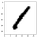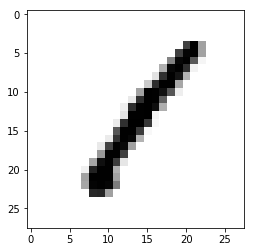I am using Sequential model and used Flatten layer to convert tensors into arrays. Using relu activation(REctified Linear Units) with different input image parameters, I degraded features vectors to 64. Lastly, I used Softmax function with 10 output entries (0 to 9). I compiled model with adam optimzer and used loss function as sparse_categorical_crossentropy. At the end I trained model using data with 2 epochs. Epoch is training loop (forward and backward) to train model.

model = tf.keras.models.Sequential() _# creating Sequential model_
model.add(tf.keras.layers.Flatten()) _# flattening the input arrays_
model.add(tf.keras.layers.Dense(128, activation=tf.nn.relu)) _# using relu activation function_
model.add(tf.keras.layers.Dense(64, activation=tf.nn.relu)) _# using relu activation function_
model.add(tf.keras.layers.Dense(10, activation=tf.nn.softmax)) _# activation function to get number of output_

model.compile(optimizer='adam', loss='sparse_categorical_crossentropy', metrics=['accuracy']) _# compiling model_

model.fit(X_train, Y_train.values, epochs=5) _# training model and fitting data_model.summary()


Now, splitting the sets.

_# splitting data to evalueate model_
X_train, X_val, Y_train, Y_val = train_test_split(X_train,
Y_train,
test_size=0.20,
random_state=42,
shuffle=True,
stratify=Y_train)


Finding the performance of the model.

val_loss , val_accuracy = model.evaluate(X_val, Y_val) _# evaluating performance of the model_
print(val_loss, val_accuracy)predictions = model.predict([X_val])
displayImageWithPredictedValue(X_val, predictions)


Output: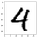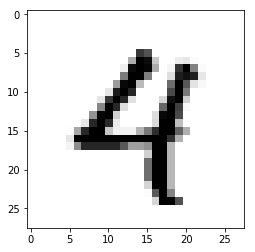test_df = test_df.values.reshape(-1,28,28,1)/255

Now finding the performance of the model.

predictions = model.predict([test_df])
displayImageWithPredictedValue(test_df, predictions)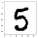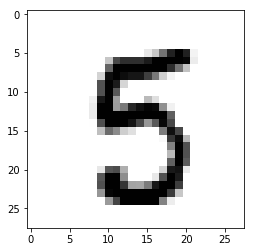# 4. Conclusion:

I created a model that can predict the correct number values from the images with accuracy 0.96614. This dataset can be further explored by applying classification methods such as SVM and K-nearest neighbours.

You can follow me on Kaggle and upvote this kernel to help others to learn it. Thank you :)

You can clap for this blog, share it and also make comments on it.